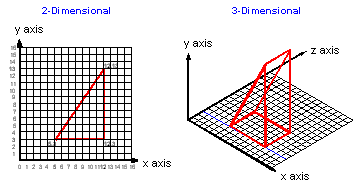# x-y-z matrix

Also found in: Wikipedia.

## x-y-z matrix

A three-dimensional structure. The x-axis and y-axis represent the first two dimensions; the z-axis, the third dimension. In a graphic image, the x and y denote width and height; the z denotes depth.Site: Follow: Share:
Open / Close